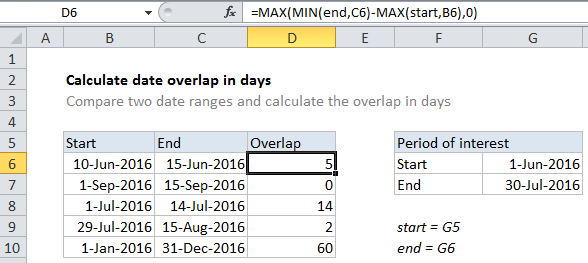## Excel Office

Excel How Tos, Tutorials, Tips & Tricks, Shortcuts

# Calculate date overlap in days in Excel

If you need to calculate the number of days that overlap in two date ranges, then this tutorials is for you.

You can use basic date arithmetic, together with the the MIN and MAX functions. See example below;

Worked Example:   Minimum value if in Excel

## Formula

`=MAX(MIN(end1,end2)-MAX(start1,start2)+1,0)`## Explanation

In the example shown, the formula in D6 is:

`=MAX(MIN(end,C6)-MAX(start,B6)+1,0)`

### How this formula works

Excel dates are just serial numbers, so you can calculate durations by subtracting the earlier date from the later date.

This is what happens at the core of the formula here:

`MIN(end,C6)-MAX(start,B6)+1`

Here are are simply subtracting an earlier date from a later date. To figure out what dates to use for each date range comparison, we use MIN to get the earliest end date, and MAX to get the latest end date.

Worked Example:   nth largest value with duplicates in Excel

Finally, we use the MAX function to trap negative values and return zero instead. Using MAX this way is a clever way to avoid using IF.

Worked Example:   Larger of two values in Excel